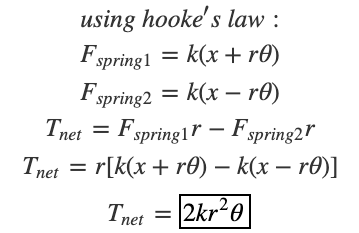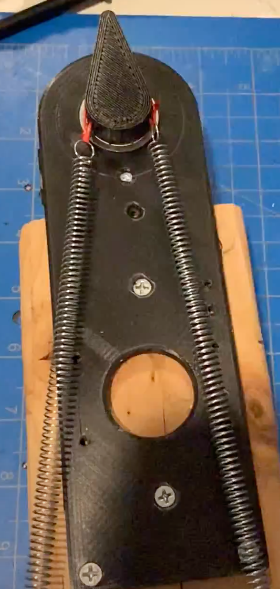Search
• Sajiv Shah

# Testing a Hypothesis - Changing Stiffness by Extending Springs

Updated: 7 days agoThe image above is from a paper that was published in the 2018 International Conference for Robotics and Automation (ICRA). This image is from a clip where the author states that this mechanism, which is meant to resemble a human joint, achieves variable stiffness. He says that when one spring is extended (squiggly lines) , the joint is rotated, but if both springs are extended, the joint becomes stiffer.

That one sentence contained lots of value as it brings up a method for stiffness modulation that is worth investigating considering it's simplicity. Let's look at what happens when springs are placed in the way described above.hmmm...this looks a awful lot like the Series Elastic Actuator we talked about earlier

In this setup, we have the springs setup so they are creating tension in the belt between the motor and joint. There is also an additional component that can pull the motor away from the joint, which in other words, stretches both springs as described by the author of the paper above. One measure of the variable stiffness on an actuator is the output torque that it applies at the joint. Let's calculate the output torque as a function of the initial spring extension, x.Under the assumption that this spring system is linear (this will be important to note in the future) it appears that the net torque on this actuator is is no way related to the initial extension x. The terms containing x cancel out in the second-to-last step of the math above, which also makes intuitive sense considering the fact that applying bigger forces on opposing sides of a joint has no effect.

Although the math shows that this hypothesis is not theoretically correct, we want to confirm this experimentally, so I designed the test stands below and used a force scale to measure how different extensions play a role on the torque applied at the end.The reason I built two tests stands is because I was surprised by the results from the first. Changing the initial extension actually varied the torque value of the end effector.

But how could this be? What is our math missing? Or what is error in the experiment?

See All